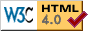# Spatial Analysis and Decision Assistance## Geospatial Analysis (Simulation)

Geostatistical simulation allows users to create multiple realizations of what a spatially distributed attribute (contamination) might look like given the data at hand and the model selection. By realizations, we mean maps. So if there are 1000 simulations, SADA produces 1000 individual equiprobable maps of how an attribute (contamination) might be distributed. In the following image three simulations taken from a larger set are presetned.Simulation has a couple of advantages over standard interpolation. First the results tend to be more heterogenous, a result that is more often than not observed in real environmental data. Secondly, the results provide estimates of local uncertainty (meaning uncertainty about the concentration at any given point) and joint uncertainty (meaning uncertainty about how a region of values is behaving together).

Simulations can be used as a stochastic input to an outside model such as a groundwater model. For example, one might simulate the source term or the soil porosity or some other important term. Each simulation serves as a single input into the end model which then in turn produces a single outcome. Multiple simualtions then yield multiple outcomes and one can quantify uncertainty in the external model (e.g. groundwater model) as a function of uncertainty in the spatial variation of the input data.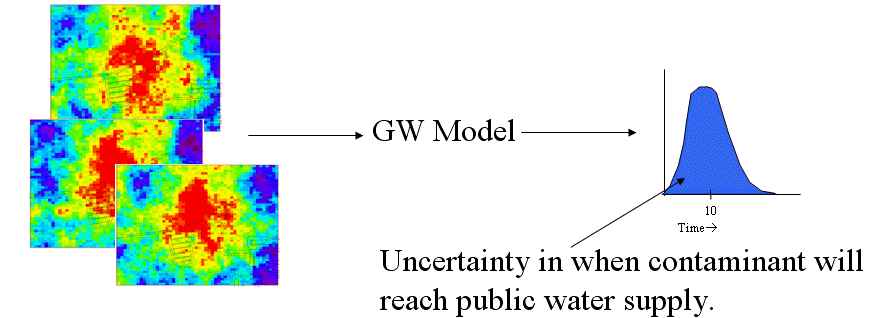Simulations can also be post processed in a variety of ways. One can average the simulations at each node to produce a contour map, evaluated simulations results at each node to determine the probability of exceeding a decision criteria to produce a probability map, joint probablity maps, and calculate the simulation variances at each node.

### Simulation Averages (contour)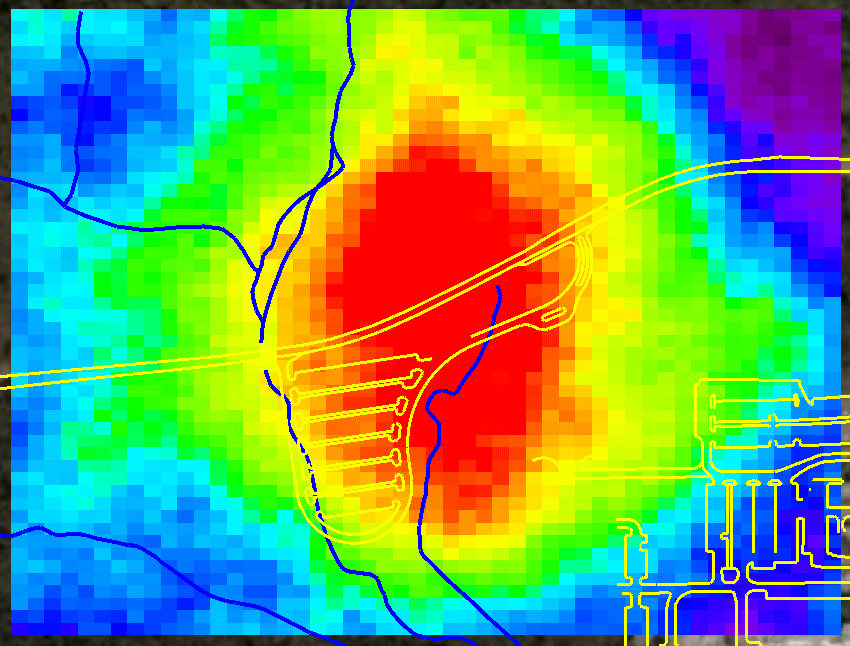### Probability of Exceeding (e.g. probability any node exceeds 3pCi/g)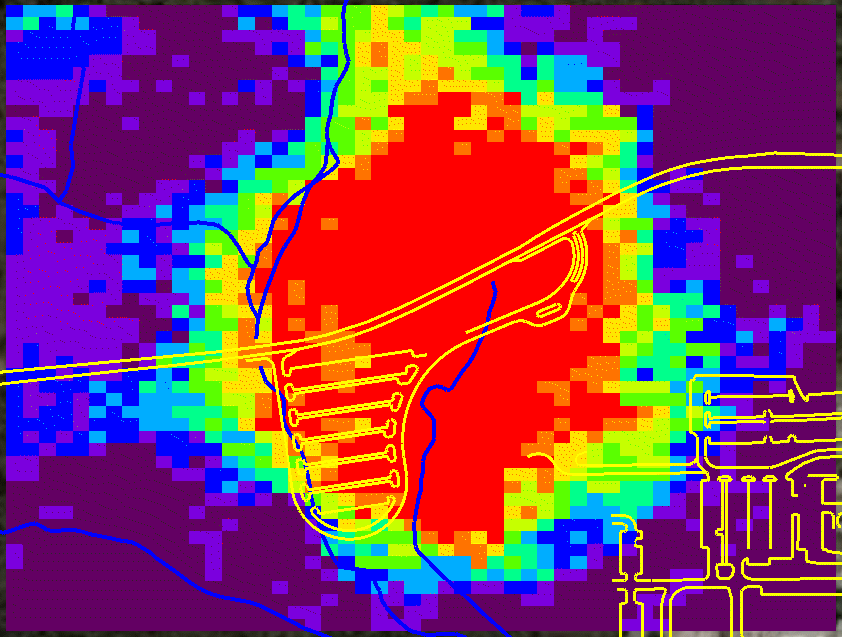### Joint Probability of Exceeding(e.g. probability that a group of nodes exceed 3pCi/g at the same time)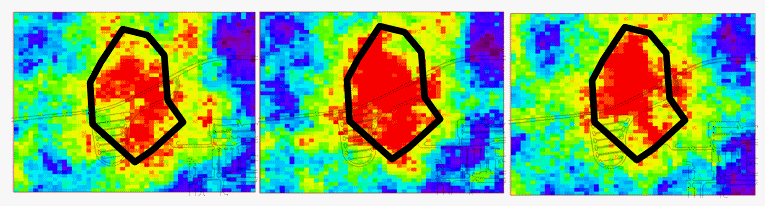### Simulation Variance